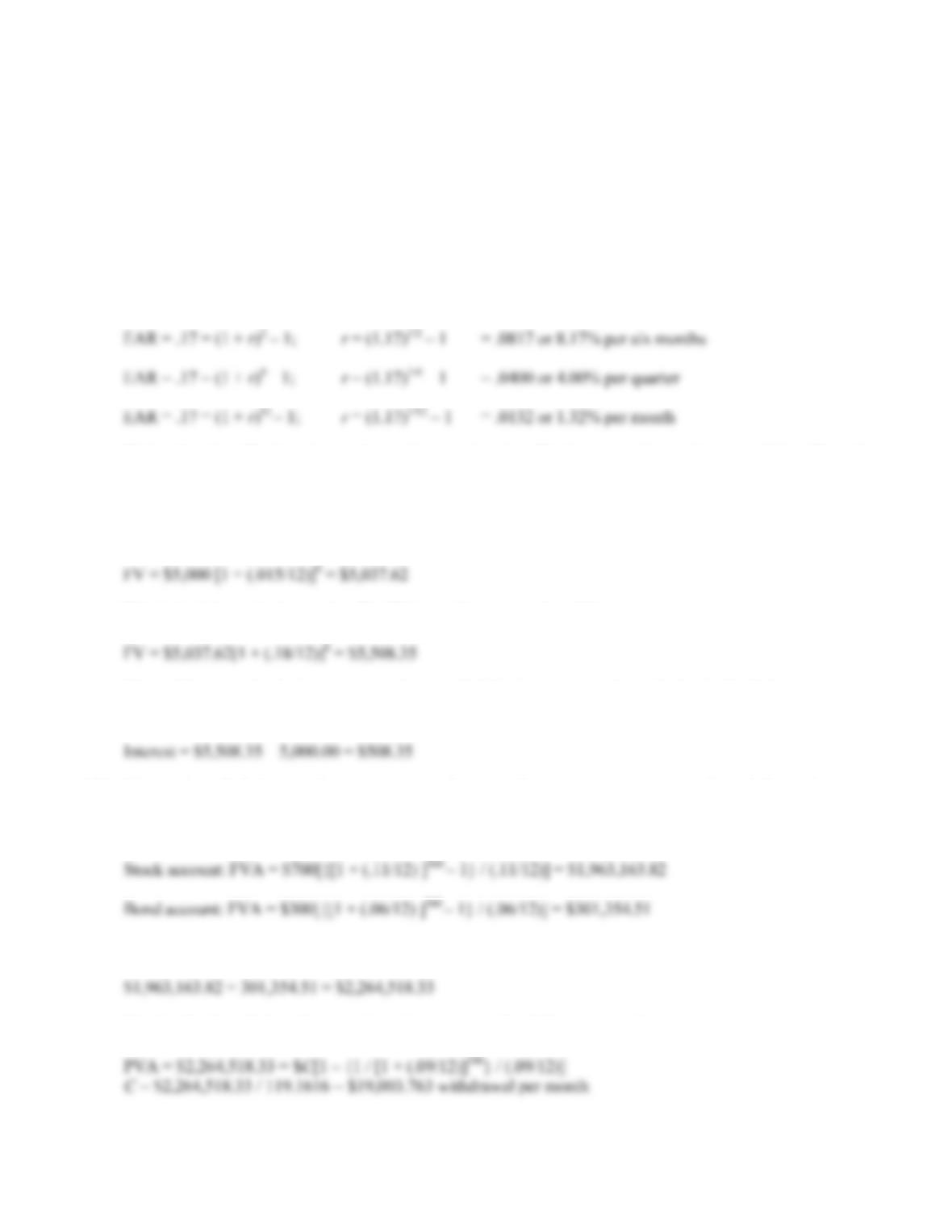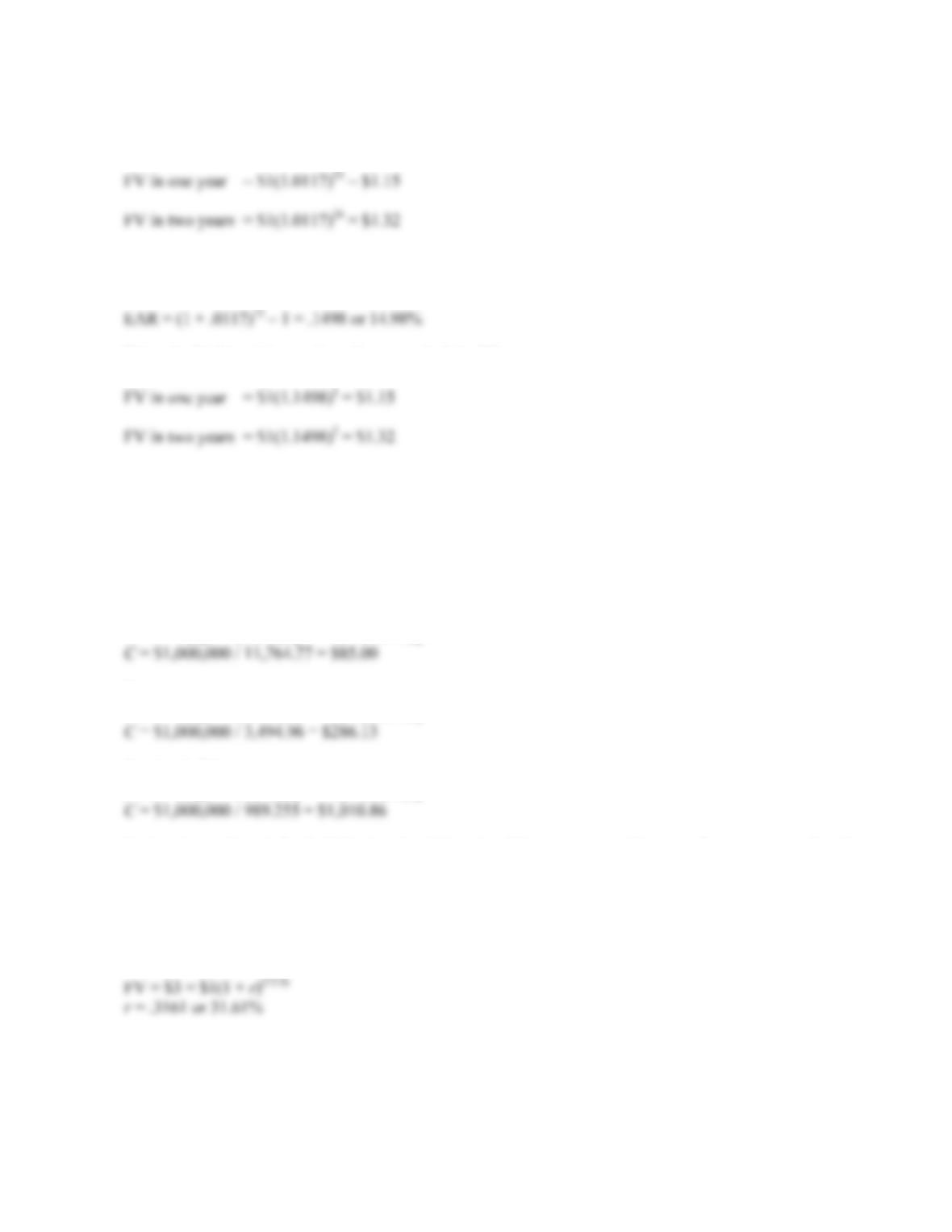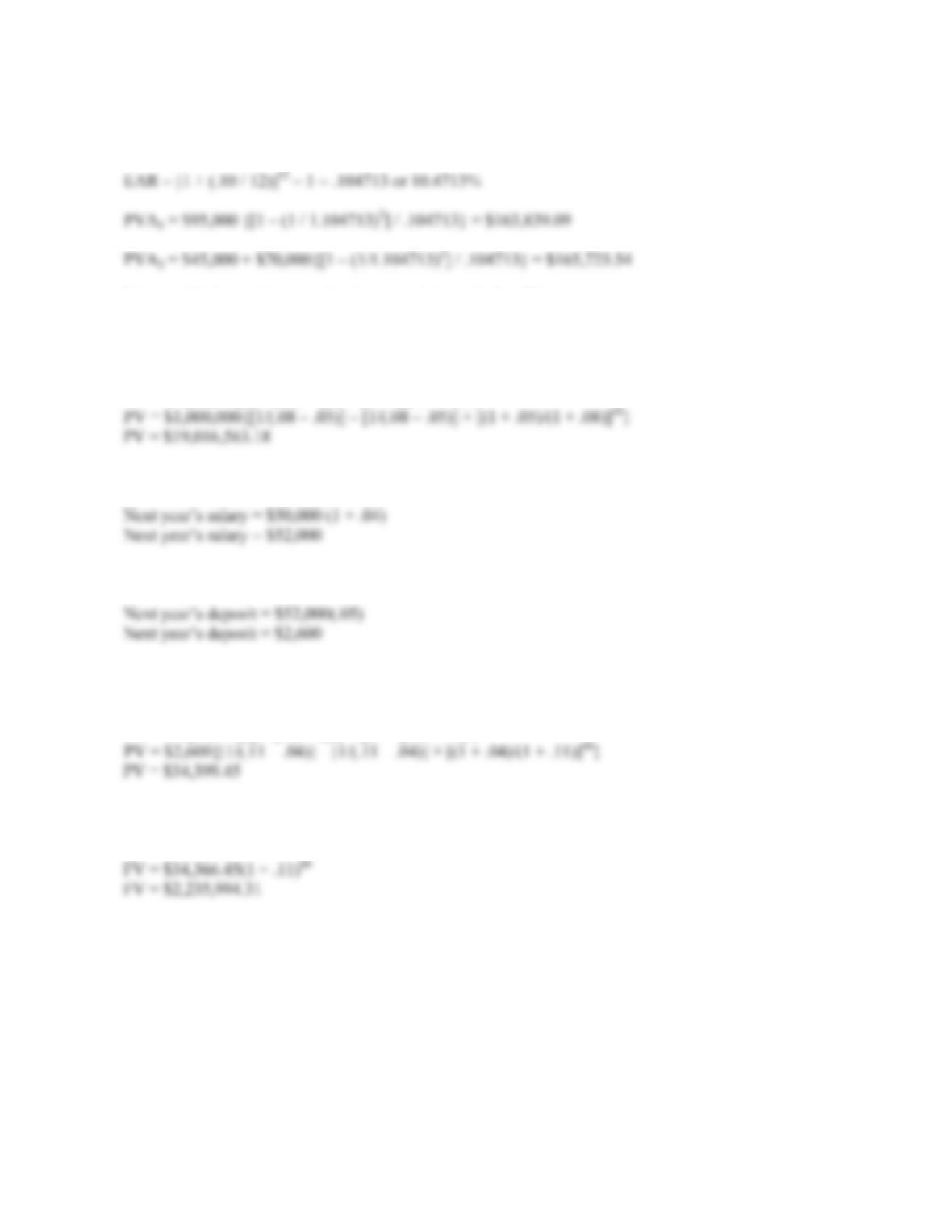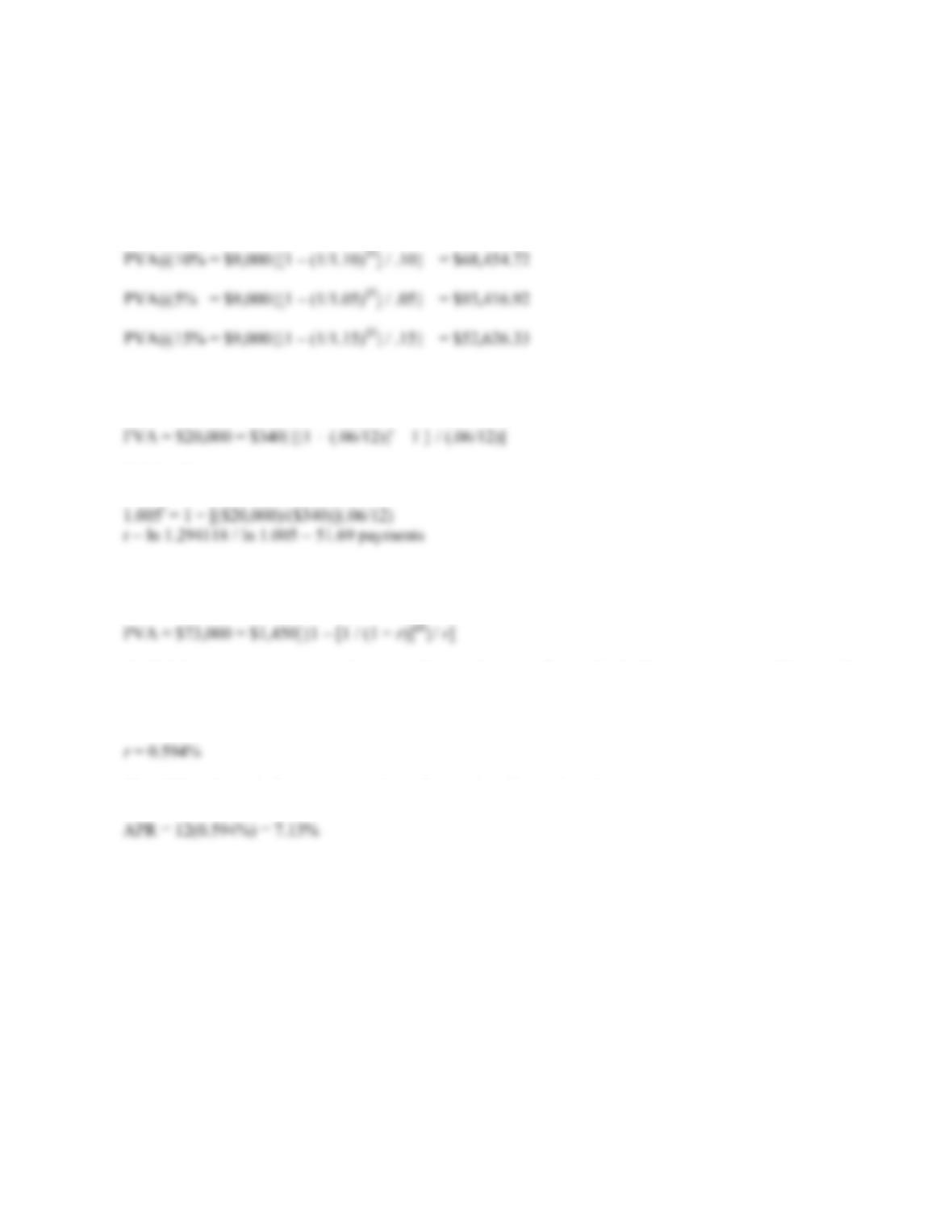Type
Quiz
Book Title
Fundamentals of Corporate Finance Standard Edition 9th Edition
ISBN 13
978-0073382395

### 978-0073382395 Chapter 6 Questions and Problems 32-41

April 3, 2019CHAPTER 6 B-77
Setting the two equal, we get:
(.07)(10) = (1 + r)10 – 1
r = 1.71/10 – 1 = .0545 or 5.45%
30. Here we need to convert an EAR into interest rates for different compounding periods. Using the equation
for the EAR, we get:
EAR = [1 + (APR / m)]m – 1
Notice that the effective six month rate is not twice the effective quarterly rate because of the effect of
compounding.
31. Here we need to find the FV of a lump sum, with a changing interest rate. We must do this problem in two
parts. After the first six months, the balance will be:
This is the balance in six months. The FV in another six months will be:
The problem asks for the interest accrued, so, to find the interest, we subtract the beginning balance
from the FV. The interest accrued is:
32. We need to find the annuity payment in retirement. Our retirement savings ends and the retirement
withdrawals begin, so the PV of the retirement withdrawals will be the FV of the retirement savings. So,
we find the FV of the stock account and the FV of the bond account and add the two FVs.
So, the total amount saved at retirement is:
Solving for the withdrawal amount in retirement using the PVA equation gives us:B-78 SOLUTIONS
33. We need to find the FV of a lump sum in one year and two years. It is important that we use the
number of months in compounding since interest is compounded monthly in this case. So:
There is also another common alternative solution. We could find the EAR, and use the number of years as
our compounding periods. So we will find the EAR first:
Using the EAR and the number of years to find the FV, we get:
Either method is correct and acceptable. We have simply made sure that the interest compounding period is
the same as the number of periods we use to calculate the FV.
34. Here we are finding the annuity payment necessary to achieve the same FV. The interest rate given is a 12
percent APR, with monthly deposits. We must make sure to use the number of months in the equation. So,
using the FVA equation:
Starting today:
FVA = C[{[1 + (.12/12) ]480 – 1} / (.12/12)]
Starting in 10 years:
FVA = C[{[1 + (.12/12) ]360 – 1} / (.12/12)]
Starting in 20 years:
FVA = C[{[1 + (.12/12) ]240 – 1} / (.12/12)]
Notice that a deposit for half the length of time, i.e. 20 years versus 40 years, does not mean that the
annuity payment is doubled. In this example, by reducing the savings period by one-half, the deposit
necessary to achieve the same ending value is about twelve times as large.
35. Since we are looking to quadruple our money, the PV and FV are irrelevant as long as the FV is three times
as large as the PV. The number of periods is four, the number of quarters per year. So:CHAPTER 6 B-79
36. Since we have an APR compounded monthly and an annual payment, we must first convert the interest rate
to an EAR so that the compounding period is the same as the cash flows.
You would choose the second option since it has a higher PV.
37. We can use the present value of a growing perpetuity equation to find the value of your deposits today.
Doing so, we find:
PV = C {[1/(rg)] – [1/(rg)] × [(1 + g)/(1 + r)]t}
38. Since your salary grows at 4 percent per year, your salary next year will be:
This means your deposit next year will be:
Since your salary grows at 4 percent, you deposit will also grow at 4 percent. We can use the present value
of a growing perpetuity equation to find the value of your deposits today. Doing so, we find:
PV = C {[1/(rg)] – [1/(rg)] × [(1 + g)/(1 + r)]t}
Now, we can find the future value of this lump sum in 40 years. We find:
FV = PV(1 + r)t
This is the value of your savings in 40 years.B-80 SOLUTIONS
39. The relationship between the PVA and the interest rate is:
PVA falls as r increases, and PVA rises as r decreases
FVA rises as r increases, and FVA falls as r decreases
The present values of \$9,000 per year for 10 years at the various interest rates given are:
40. Here we are given the FVA, the interest rate, and the amount of the annuity. We need to solve for the
number of payments. Using the FVA equation:
Solving for t, we get:
41. Here we are given the PVA, number of periods, and the amount of the annuity. We need to solve for the
interest rate. Using the PVA equation:
To find the interest rate, we need to solve this equation on a financial calculator, using a spreadsheet, or by
trial and error. If you use trial and error, remember that increasing the interest rate lowers the PVA, and
decreasing the interest rate increases the PVA. Using a spreadsheet, we find:
The APR is the periodic interest rate times the number of periods in the year, so: